# Chapter 10 Liquids and Solids Solids vs Liquids

• Slides: 73Chapter 10 Liquids and Solids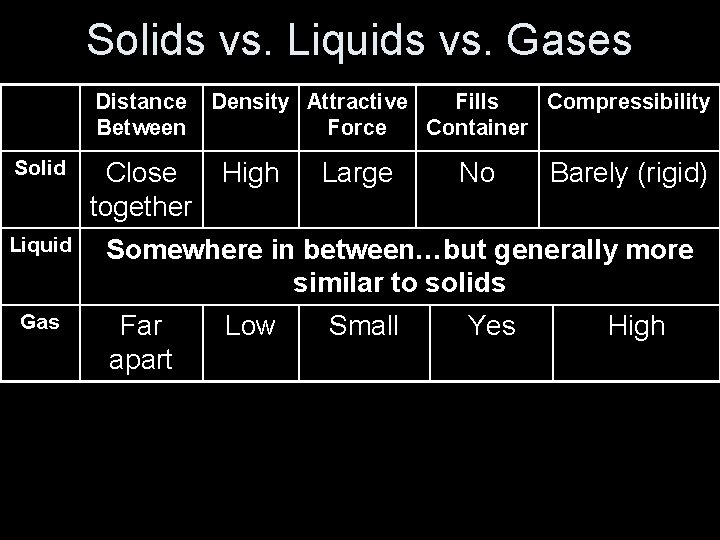Solids vs. Liquids vs. Gases Distance Between Solid Liquid Gas Close together Density Attractive Fills Compressibility Force Container High Large No Barely (rigid) Somewhere in between…but generally more similar to solids Far apart Low Small Yes HighIntermolecular Forces • Occur between (not within) molecules • Covalent/Ionic Bonding • Dipole-Dipole • London Dispersion ForcesImportant to Note • Changing states is a PHYSICAL (not chemical) change • The molecules do NOT break apart in a physical (phase) change!Dipole-Dipole Forces • Two molecules with dipoles (polarity) can have an attraction to one another called a dipole attraction • This will make the most +, - attractions and -, and +, + repulsions • Hydrogen bonding is a common (stronger) example Dipole-Dipole < Covalent < Ionic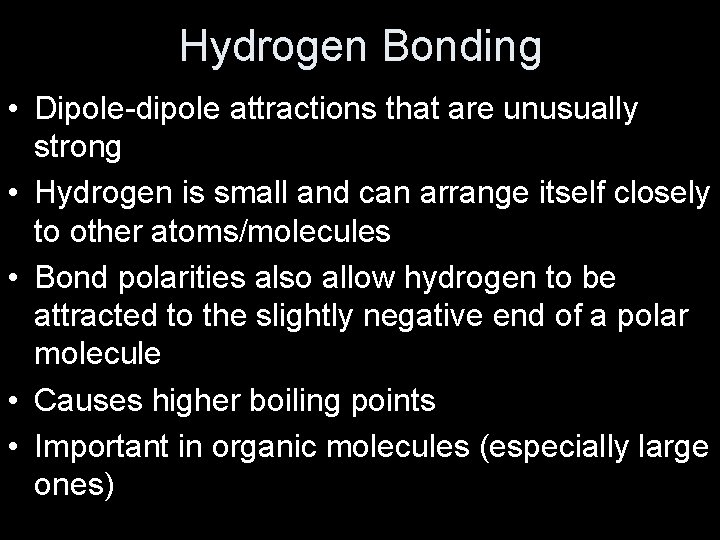Hydrogen Bonding • Dipole-dipole attractions that are unusually strong • Hydrogen is small and can arrange itself closely to other atoms/molecules • Bond polarities also allow hydrogen to be attracted to the slightly negative end of a polar molecule • Causes higher boiling points • Important in organic molecules (especially large ones)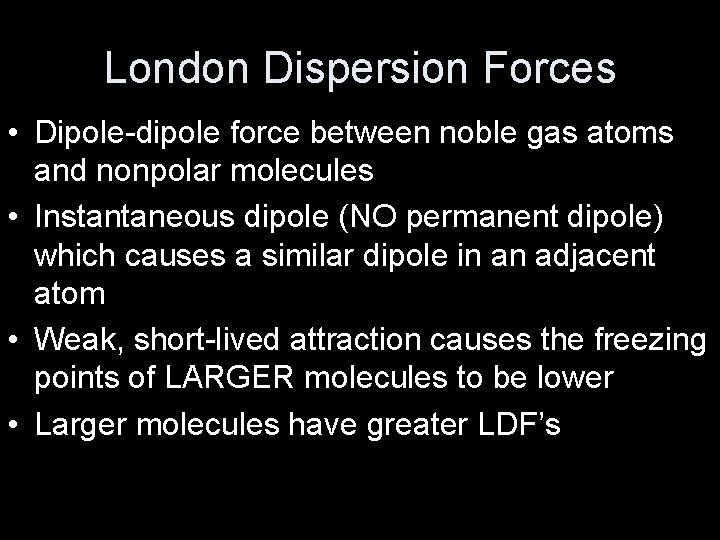London Dispersion Forces • Dipole-dipole force between noble gas atoms and nonpolar molecules • Instantaneous dipole (NO permanent dipole) which causes a similar dipole in an adjacent atom • Weak, short-lived attraction causes the freezing points of LARGER molecules to be lower • Larger molecules have greater LDF’s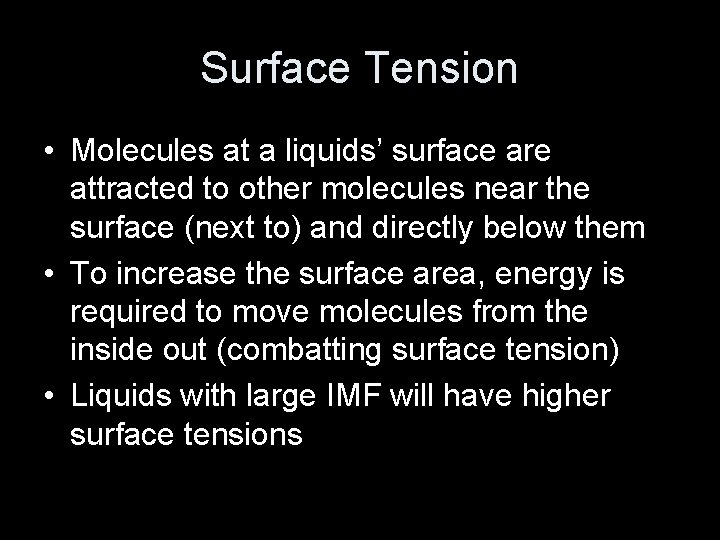Surface Tension • Molecules at a liquids’ surface are attracted to other molecules near the surface (next to) and directly below them • To increase the surface area, energy is required to move molecules from the inside out (combatting surface tension) • Liquids with large IMF will have higher surface tensions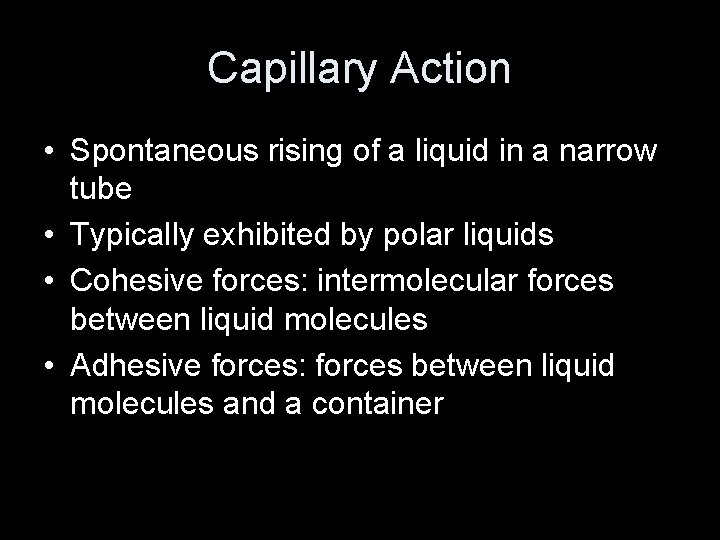Capillary Action • Spontaneous rising of a liquid in a narrow tube • Typically exhibited by polar liquids • Cohesive forces: intermolecular forces between liquid molecules • Adhesive forces: forces between liquid molecules and a container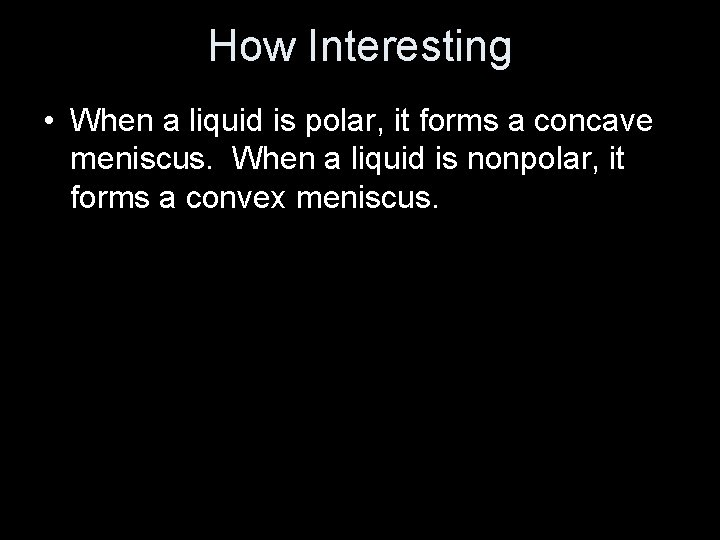How Interesting • When a liquid is polar, it forms a concave meniscus. When a liquid is nonpolar, it forms a convex meniscus.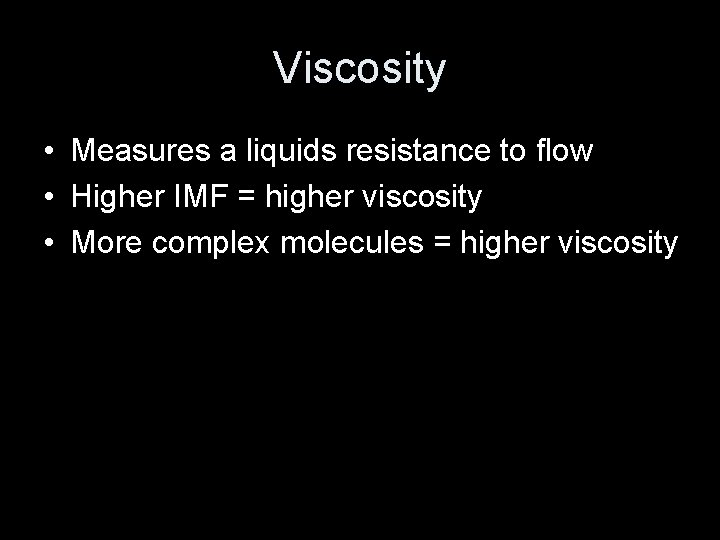Viscosity • Measures a liquids resistance to flow • Higher IMF = higher viscosity • More complex molecules = higher viscosityModeling • Solids and gases are easy to model • Liquids are not… • Spectroscopy is used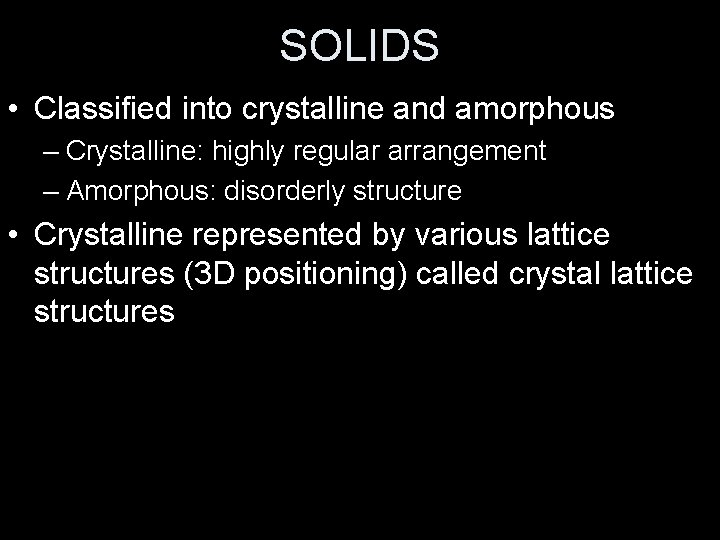SOLIDS • Classified into crystalline and amorphous – Crystalline: highly regular arrangement – Amorphous: disorderly structure • Crystalline represented by various lattice structures (3 D positioning) called crystal lattice structures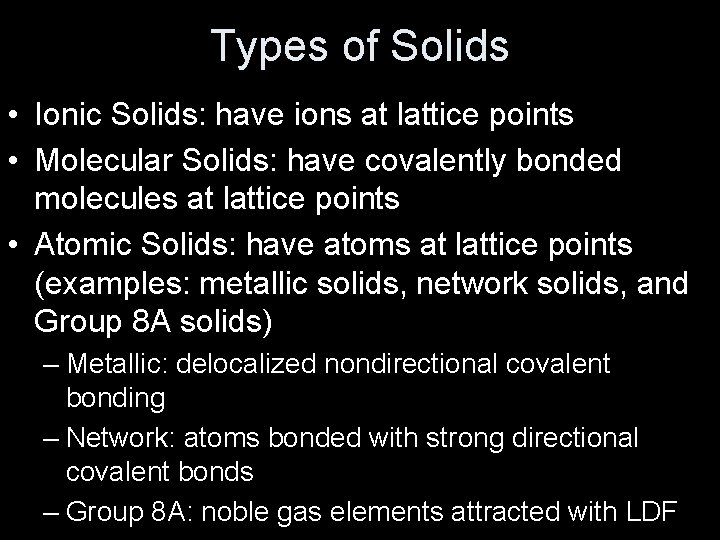Types of Solids • Ionic Solids: have ions at lattice points • Molecular Solids: have covalently bonded molecules at lattice points • Atomic Solids: have atoms at lattice points (examples: metallic solids, network solids, and Group 8 A solids) – Metallic: delocalized nondirectional covalent bonding – Network: atoms bonded with strong directional covalent bonds – Group 8 A: noble gas elements attracted with LDF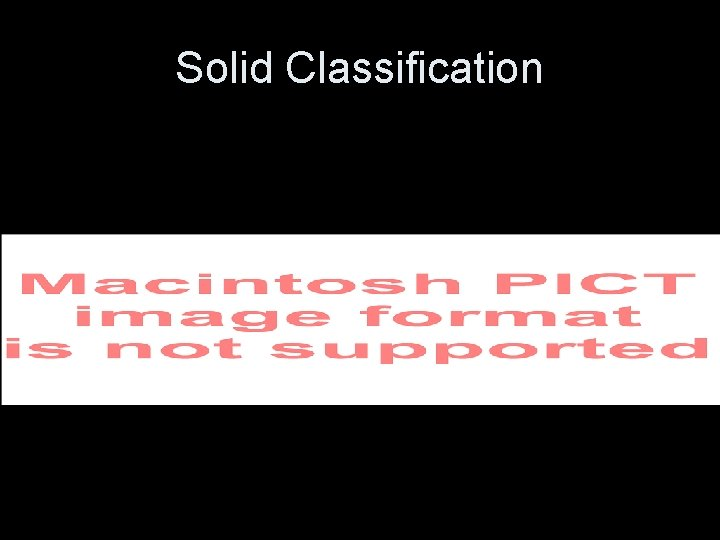Solid ClassificationPacking In Solids • Metals have characteristics based on their packing/bonding • “Closest Packing” spherical atoms are packed together in an arrangement that most efficiently uses the available space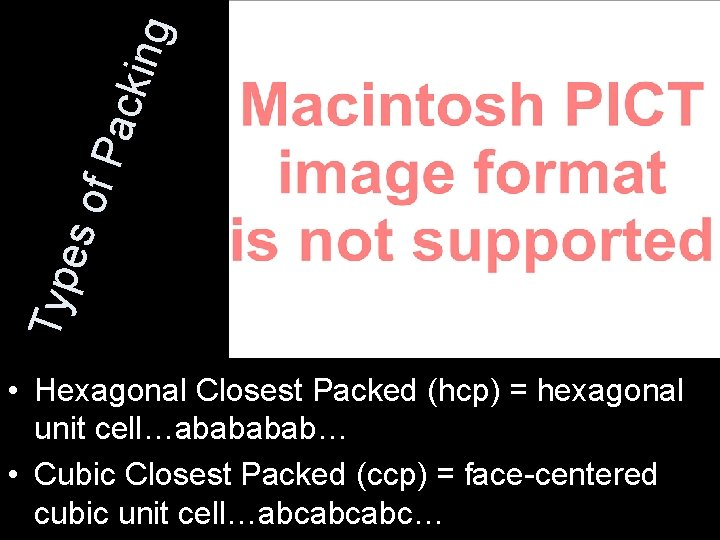king Pac es o f Typ • Hexagonal Closest Packed (hcp) = hexagonal unit cell…abab… • Cubic Closest Packed (ccp) = face-centered cubic unit cell…abcabcabc…Molecular Solids • Contain strong covalent bonding within the molecules but relatively weak forces between the molecules • The type of bond that forms between molecules will depend on the atoms present (LDF, hydrogen bonding, etc. )Ionic Solids • Stable, high-melting substances held together by strong electrostatic forces • Packing occurs so that the electrostatic forces among oppositely charged ions are maximized and repulsions are minimized • Larger ions (anions) are packed in hcp or ccp • Smaller ions (cations) are packed into holes made by the larger ions.• Trigonal holes formed by 3 spheres in one layer (smallest) • Tetrahedral holes formed by one sphere sitting in a dimple formed by three other spheres (medium) • Octahedral holes formed between two sets of three spheres (largest)Vaporization • Endothermic (requires energy) • Heat of vaporization (∆Hvap) = amount of energy required to vaporize 1 mole of a liquid at 1 atm • Liquids with high vaporization - VOLATILE • Vapor pressure is partly determined by intermolecular forces (large IMF = lower VP) • Vapor pressure increases with temperature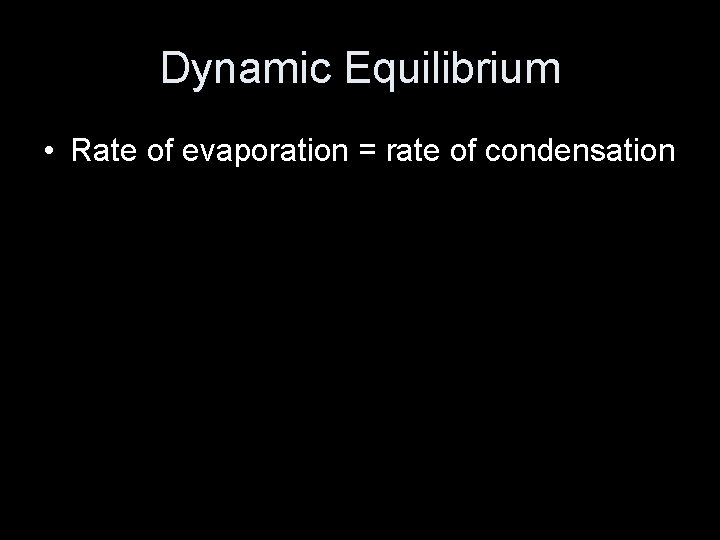Dynamic Equilibrium • Rate of evaporation = rate of condensation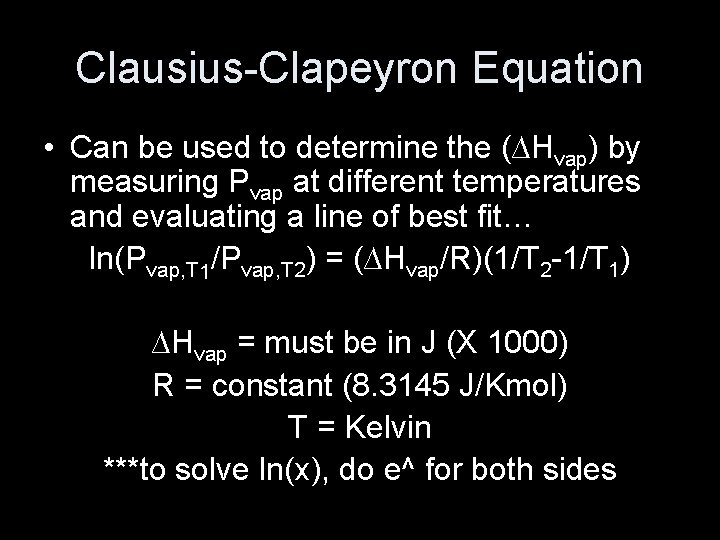Clausius-Clapeyron Equation • Can be used to determine the (∆Hvap) by measuring Pvap at different temperatures and evaluating a line of best fit… ln(Pvap, T 1/Pvap, T 2) = (∆Hvap/R)(1/T 2 -1/T 1) ∆Hvap = must be in J (X 1000) R = constant (8. 3145 J/Kmol) T = Kelvin ***to solve ln(x), do e^ for both sides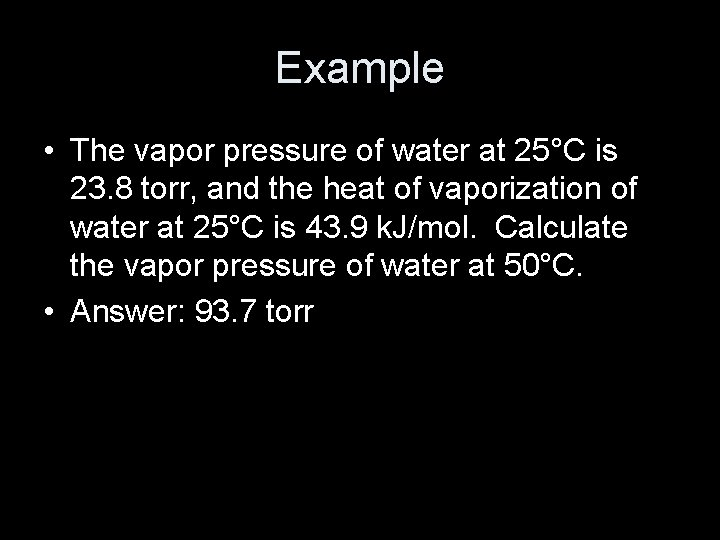Example • The vapor pressure of water at 25°C is 23. 8 torr, and the heat of vaporization of water at 25°C is 43. 9 k. J/mol. Calculate the vapor pressure of water at 50°C. • Answer: 93. 7 torr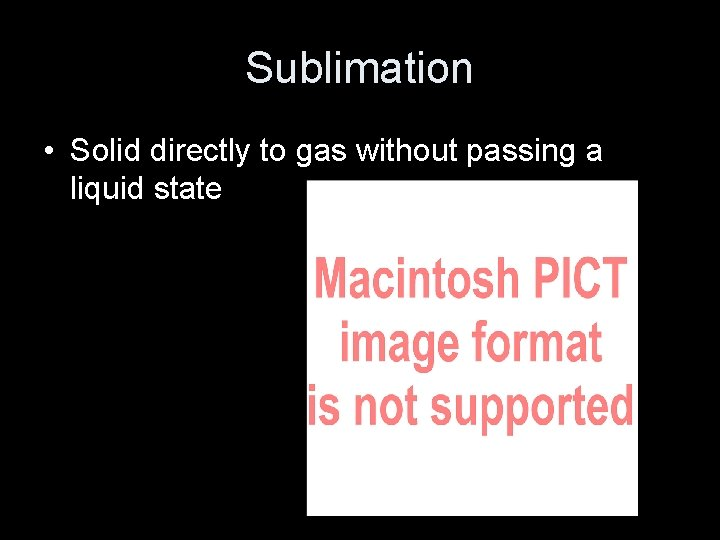Sublimation • Solid directly to gas without passing a liquid state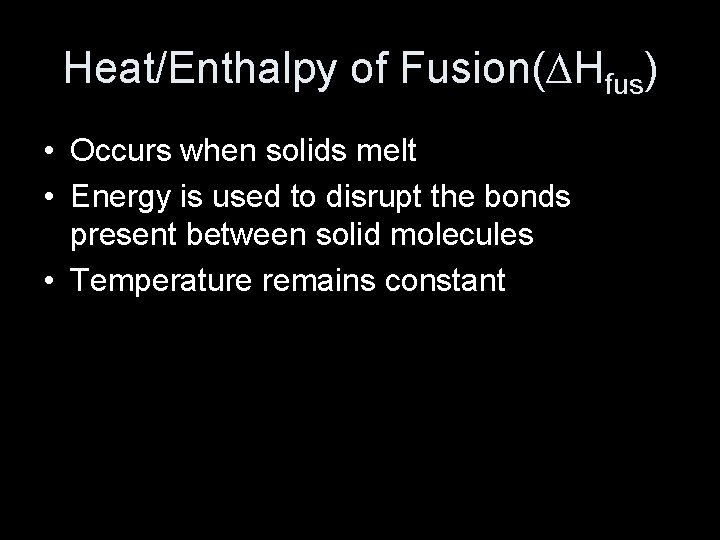Heat/Enthalpy of Fusion(∆Hfus) • Occurs when solids melt • Energy is used to disrupt the bonds present between solid molecules • Temperature remains constant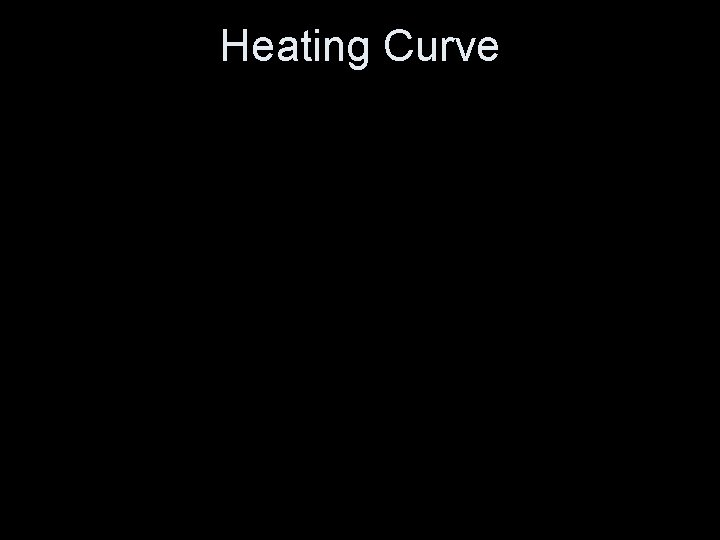Heating CurveNormal Melting/Boiling Point • Temperature at which melting/boiling occurs at STANDARD pressure (1 atm)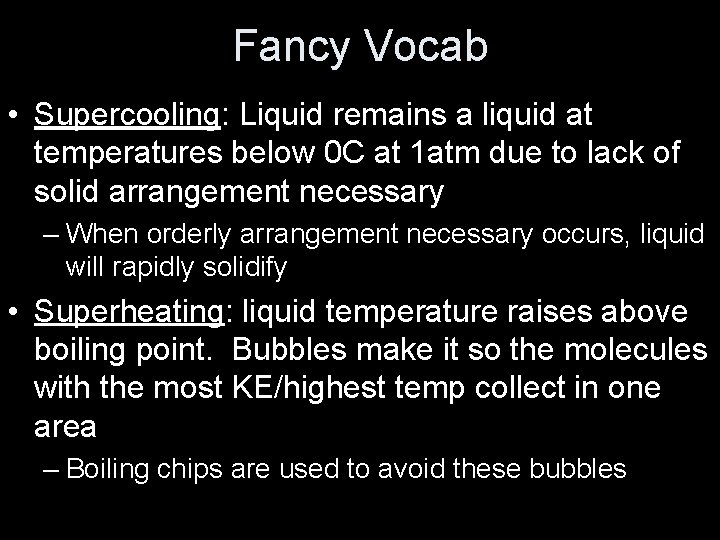Fancy Vocab • Supercooling: Liquid remains a liquid at temperatures below 0 C at 1 atm due to lack of solid arrangement necessary – When orderly arrangement necessary occurs, liquid will rapidly solidify • Superheating: liquid temperature raises above boiling point. Bubbles make it so the molecules with the most KE/highest temp collect in one area – Boiling chips are used to avoid these bubbles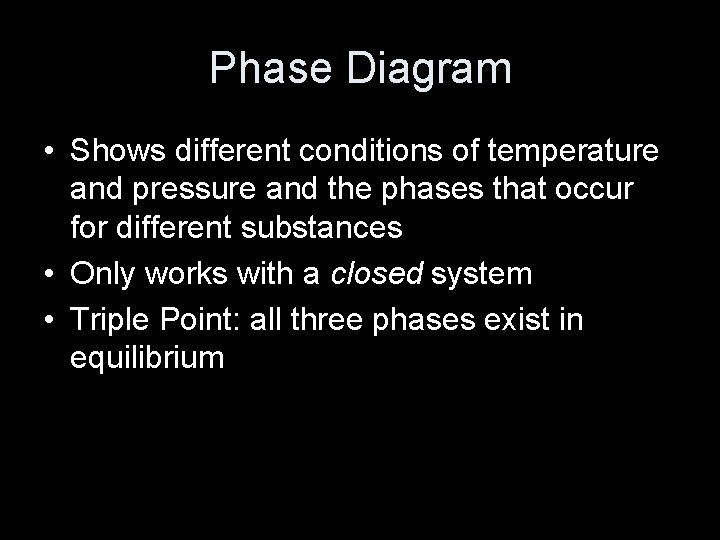Phase Diagram • Shows different conditions of temperature and pressure and the phases that occur for different substances • Only works with a closed system • Triple Point: all three phases exist in equilibrium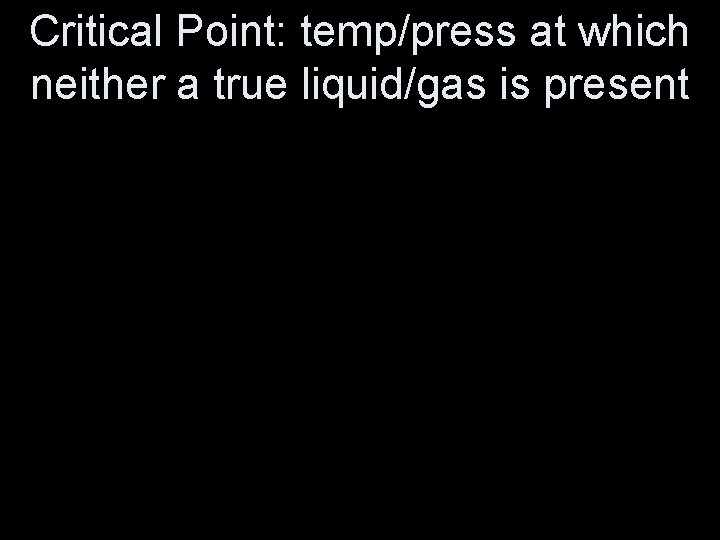Critical Point: temp/press at which neither a true liquid/gas is presentChapter 11 Properties of Solutions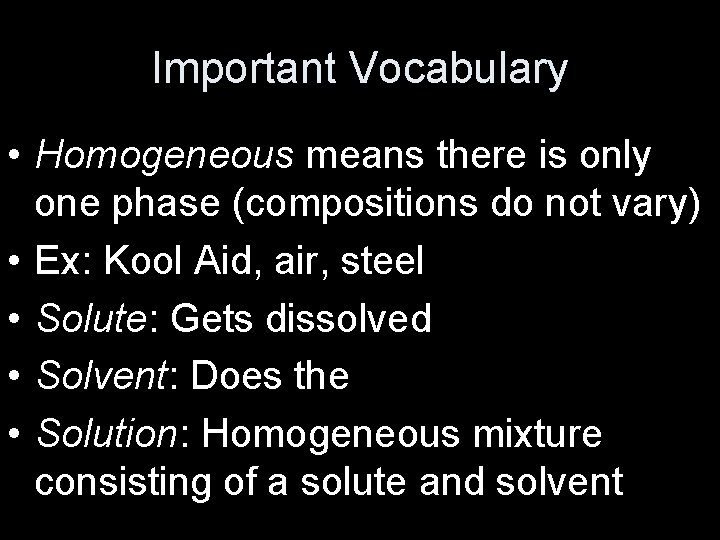Important Vocabulary • Homogeneous means there is only one phase (compositions do not vary) • Ex: Kool Aid, air, steel • Solute: Gets dissolved • Solvent: Does the dissolving • Solution: Homogeneous mixture consisting of a solute and solventDilute vs. Concentrated • Can’t be used in calculations • Molarity, mass percent, and mole fraction can be used to show solution concentrations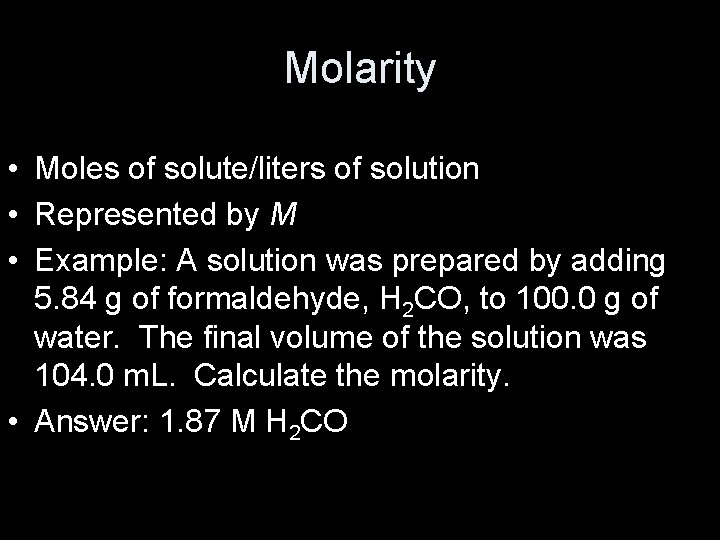Molarity • Moles of solute/liters of solution • Represented by M • Example: A solution was prepared by adding 5. 84 g of formaldehyde, H 2 CO, to 100. 0 g of water. The final volume of the solution was 104. 0 m. L. Calculate the molarity. • Answer: 1. 87 M H 2 CO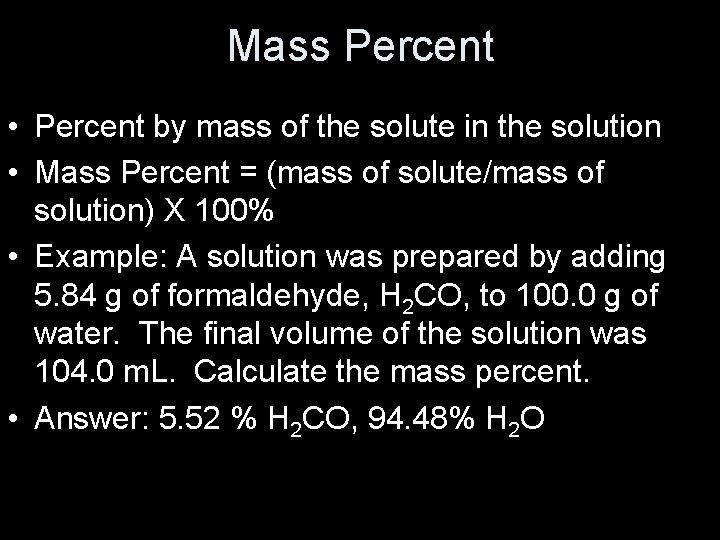Mass Percent • Percent by mass of the solute in the solution • Mass Percent = (mass of solute/mass of solution) X 100% • Example: A solution was prepared by adding 5. 84 g of formaldehyde, H 2 CO, to 100. 0 g of water. The final volume of the solution was 104. 0 m. L. Calculate the mass percent. • Answer: 5. 52 % H 2 CO, 94. 48% H 2 O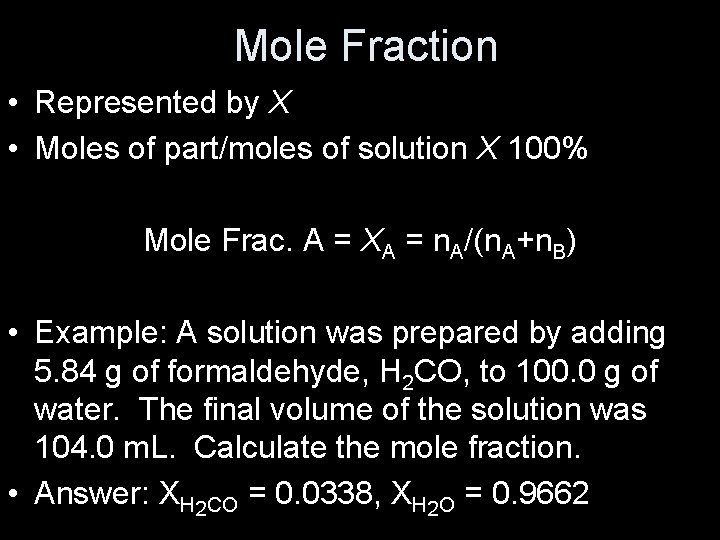Mole Fraction • Represented by X • Moles of part/moles of solution X 100% Mole Frac. A = XA = n. A/(n. A+n. B) • Example: A solution was prepared by adding 5. 84 g of formaldehyde, H 2 CO, to 100. 0 g of water. The final volume of the solution was 104. 0 m. L. Calculate the mole fraction. • Answer: XH 2 CO = 0. 0338, XH 2 O = 0. 9662Molality • Represented by m • Moles of solute per kilogram of solvent Molality = moles of solute/kilogram of solvent • Example: A solution was prepared by adding 5. 84 g of formaldehyde, H 2 CO, to 100. 0 g of water. The final volume of the solution was 104. 0 m. L. Calculate the molality. • Answer: 1. 94 m H 2 CONEW: Normality (N) • Number of “equivalents” per liter of solution • Equivalents - depends on reaction: – Acid-base reactions…mass of acid/base that can use/accept ONE mole of protons – Oxidation-reduction…quantity of oxidizing/reducing agent that will react with ONE mole of electrons • NOT ON AP EXAM!!Normality Example • Given the following reaction: H 3 PO 4 + 3 Na. OH --> PO 43 - + 3 H 2 O + 3 Na+ If we have 28. 42 g H 3 PO 4 in 800 m. L of water, what is the normality of the solution? Answer: 1. 09 N H 3 PO 4Solubility • Shows what will dissolve in what • “Like dissolves like” = polar solvents will dissolve polar/ionic solutes and nonpolar solvents will dissolve nonpolar solutesFactors Affecting Solubility 1. Structure 2. Pressure 3. Temperature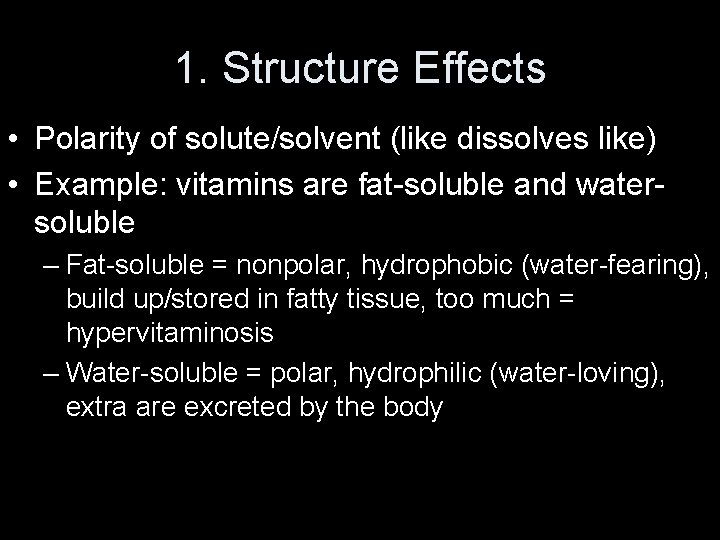1. Structure Effects • Polarity of solute/solvent (like dissolves like) • Example: vitamins are fat-soluble and watersoluble – Fat-soluble = nonpolar, hydrophobic (water-fearing), build up/stored in fatty tissue, too much = hypervitaminosis – Water-soluble = polar, hydrophilic (water-loving), extra are excreted by the body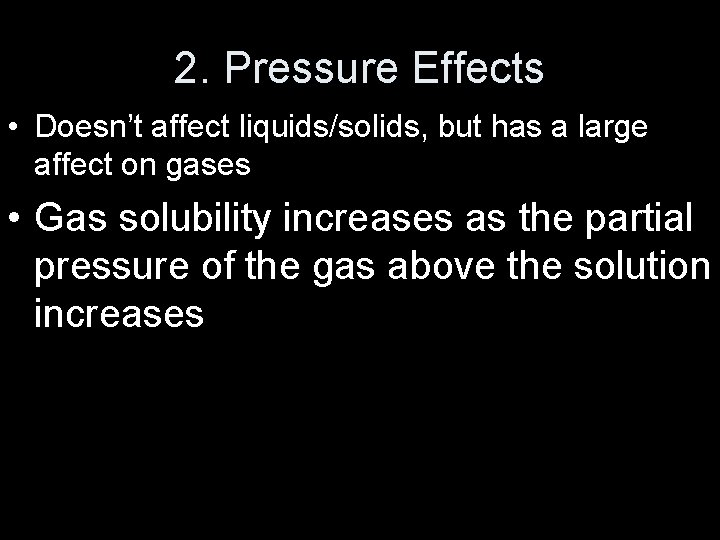2. Pressure Effects • Doesn’t affect liquids/solids, but has a large affect on gases • Gas solubility increases as the partial pressure of the gas above the solution increases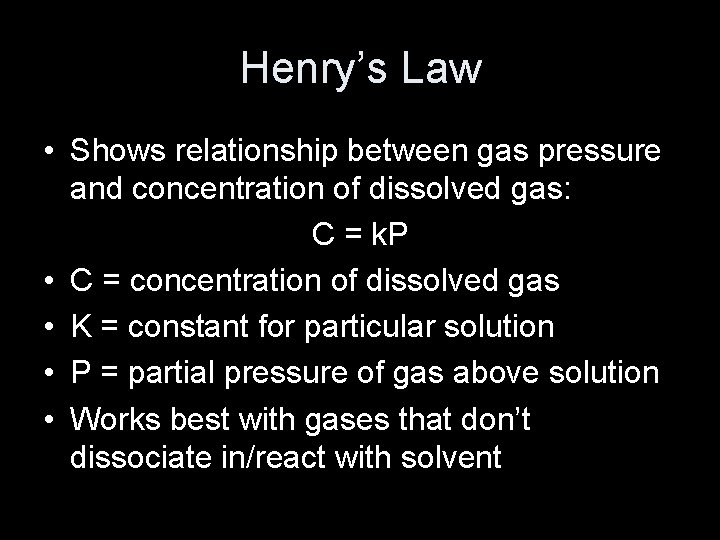Henry’s Law • Shows relationship between gas pressure and concentration of dissolved gas: C = k. P • C = concentration of dissolved gas • K = constant for particular solution • P = partial pressure of gas above solution • Works best with gases that don’t dissociate in/react with solventHenry’s Law Example • The solubility of O 2 is 2. 2 X 10 -4 M at 0 C and 0. 10 atm. Calculate the solubility of O 2 at 0 C and 0. 35 atm. • Answer: 7. 7 X 10 -4 M O 2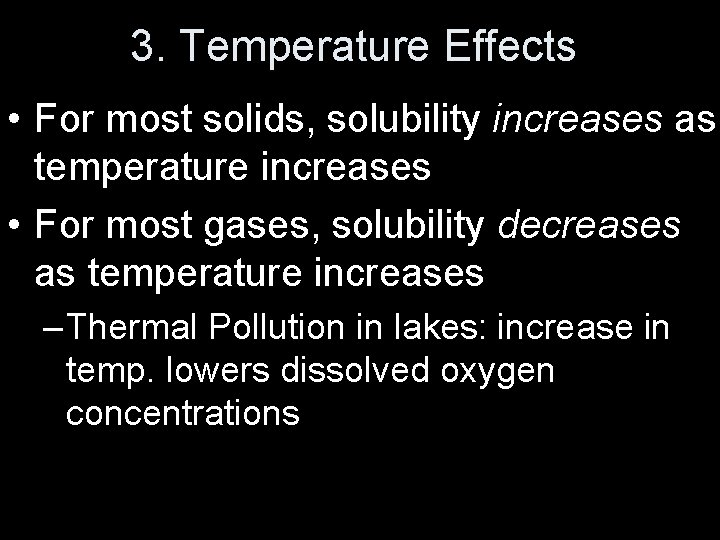3. Temperature Effects • For most solids, solubility increases as temperature increases • For most gases, solubility decreases as temperature increases – Thermal Pollution in lakes: increase in temp. lowers dissolved oxygen concentrationsVapor Pressure of Solutions • If a solution contains a nonvolitile (not easily vaporized) solute, its vapor pressure is LOWER than the pure solvent. • Shells of water solvation make it so it’s harder for the solvent to vaporize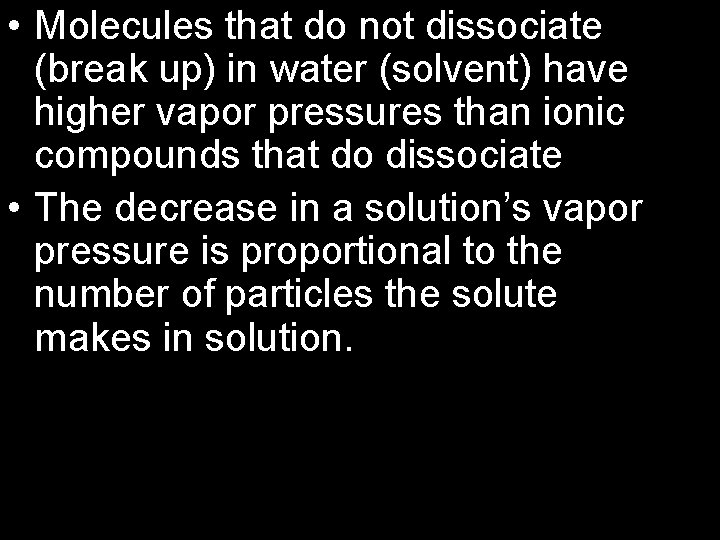• Molecules that do not dissociate (break up) in water (solvent) have higher vapor pressures than ionic compounds that do dissociate • The decrease in a solution’s vapor pressure is proportional to the number of particles the solute makes in solution.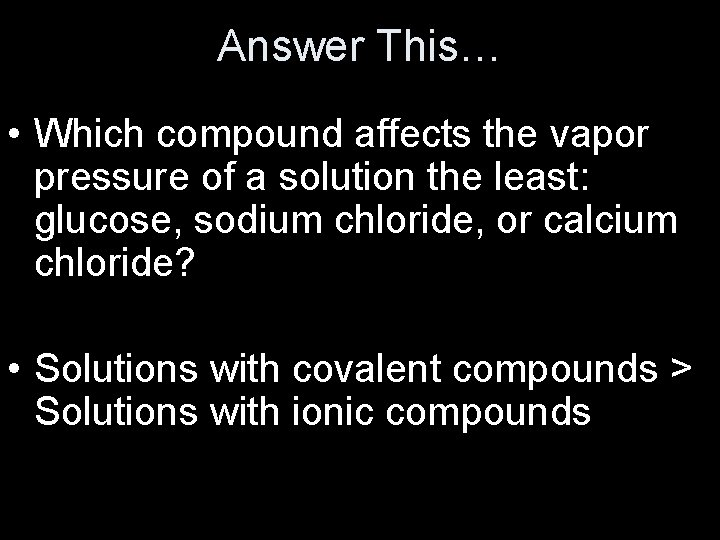Answer This… • Which compound affects the vapor pressure of a solution the least: glucose, sodium chloride, or calcium chloride? • Solutions with covalent compounds > Solutions with ionic compounds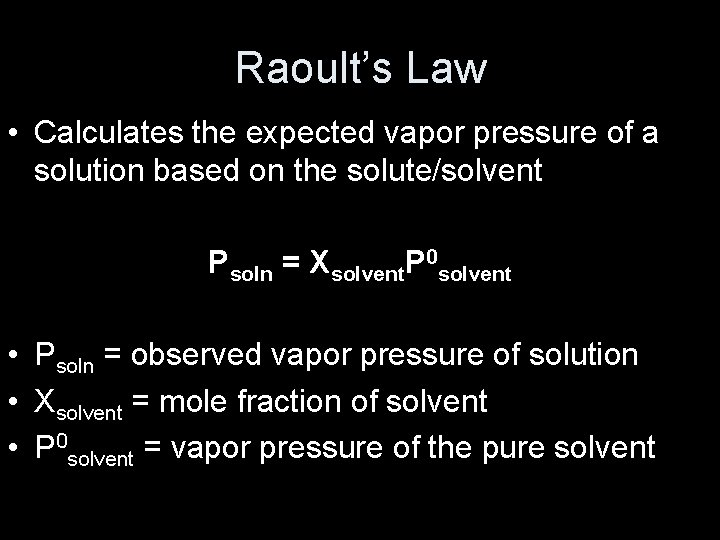Raoult’s Law • Calculates the expected vapor pressure of a solution based on the solute/solvent Psoln = Xsolvent. P 0 solvent • Psoln = observed vapor pressure of solution • Xsolvent = mole fraction of solvent • P 0 solvent = vapor pressure of the pure solventExample • Glycerin, C 3 H 8 O 3, is a nonvolatile liquid. What is the vapor pressure of a solution made by adding 164 g of glycerin to 338 m. L of H 2 O at 39. 8 C? The vapor pressure of pure water at 39. 8 C is 54. 74 torr and its density is 0. 992 g/m. L. • Answer: 50. 0 torr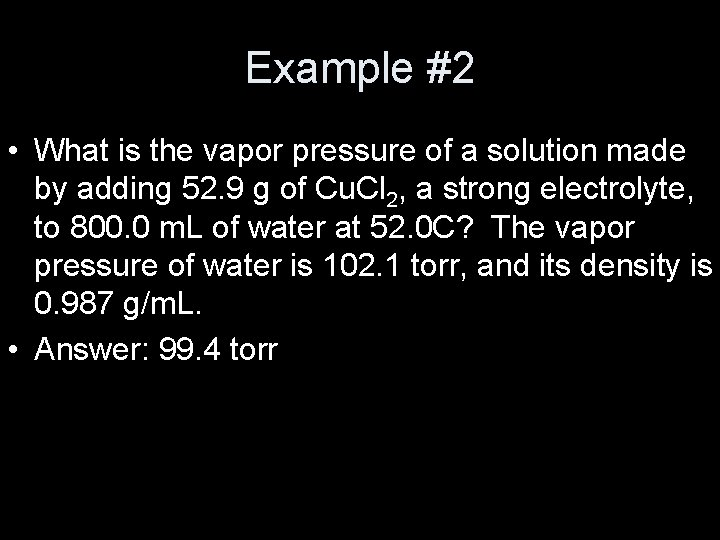Example #2 • What is the vapor pressure of a solution made by adding 52. 9 g of Cu. Cl 2, a strong electrolyte, to 800. 0 m. L of water at 52. 0 C? The vapor pressure of water is 102. 1 torr, and its density is 0. 987 g/m. L. • Answer: 99. 4 torr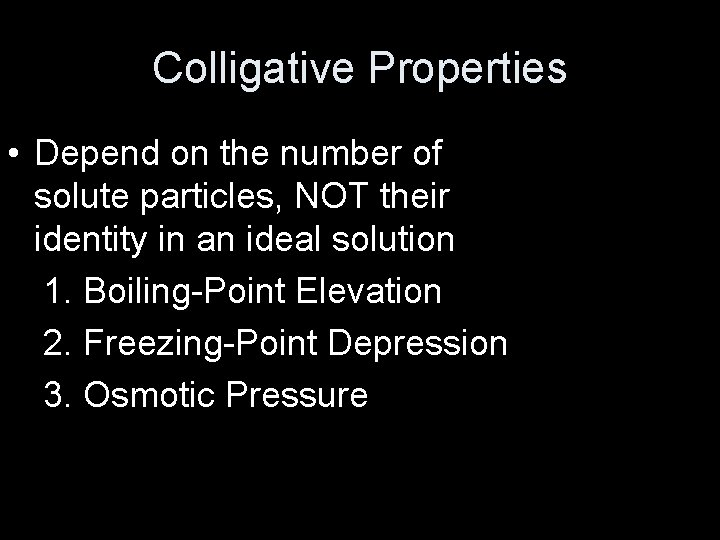Colligative Properties • Depend on the number of solute particles, NOT their identity in an ideal solution 1. Boiling-Point Elevation 2. Freezing-Point Depression 3. Osmotic Pressure1. Boiling-Point Elevation • Review boiling point definition: when vapor pressure = atmospheric pressure • When solute is added to solvent, it lowers the vapor pressure. • More kinetic energy must be added to bring the solution to boiling • Boiling point is HIGHER in solutions than in pure solvents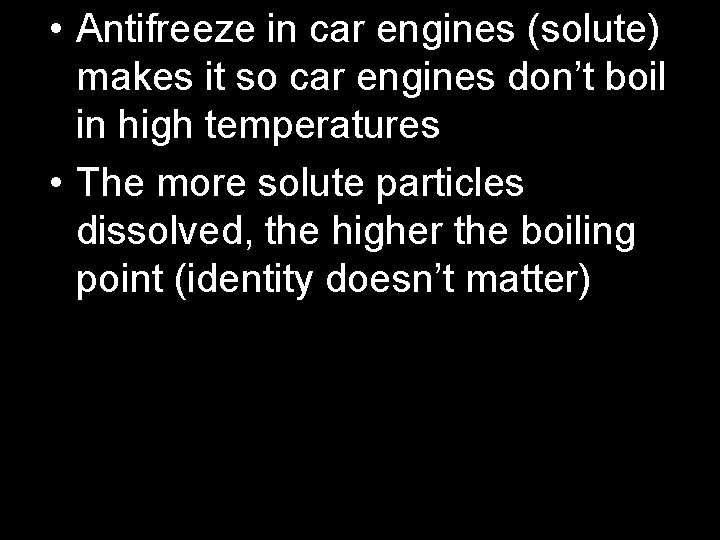• Antifreeze in car engines (solute) makes it so car engines don’t boil in high temperatures • The more solute particles dissolved, the higher the boiling point (identity doesn’t matter)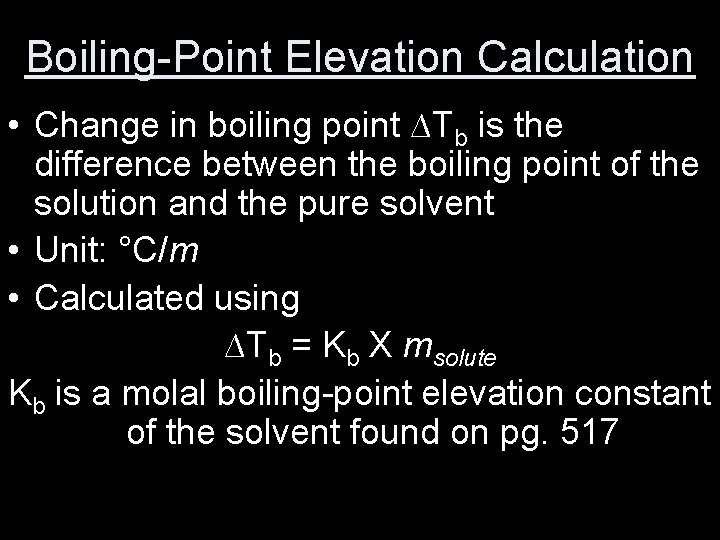Boiling-Point Elevation Calculation • Change in boiling point ∆Tb is the difference between the boiling point of the solution and the pure solvent • Unit: °C/m • Calculated using ∆Tb = Kb X msolute Kb is a molal boiling-point elevation constant of the solvent found on pg. 517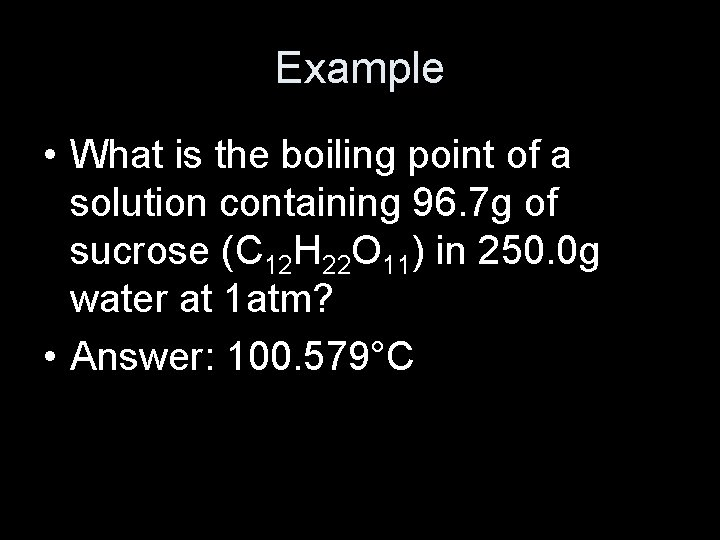Example • What is the boiling point of a solution containing 96. 7 g of sucrose (C 12 H 22 O 11) in 250. 0 g water at 1 atm? • Answer: 100. 579°C2. Freezing-Point Depression • When solute is present, the normal molecular freezing pattern is disrupted • This makes it so the solution has to lose more kinetic energy (get colder) in order to solidify • Freezing point of the solution is LOWER than that of the pure solvent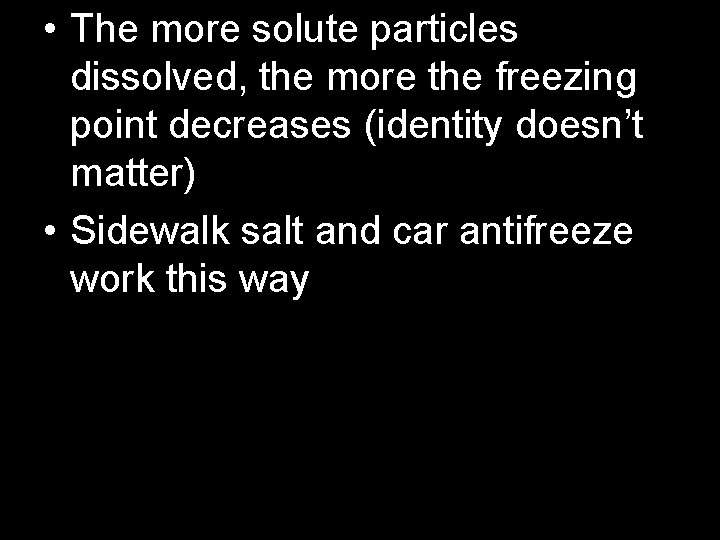• The more solute particles dissolved, the more the freezing point decreases (identity doesn’t matter) • Sidewalk salt and car antifreeze work this way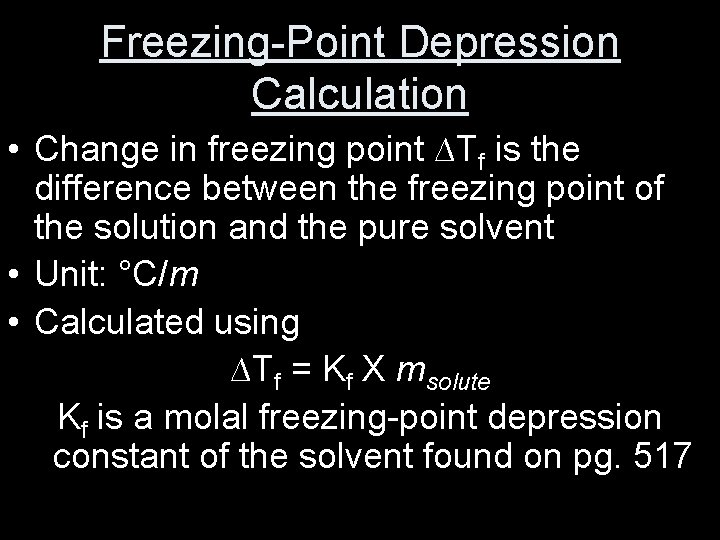Freezing-Point Depression Calculation • Change in freezing point ∆Tf is the difference between the freezing point of the solution and the pure solvent • Unit: °C/m • Calculated using ∆Tf = Kf X msolute Kf is a molal freezing-point depression constant of the solvent found on pg. 517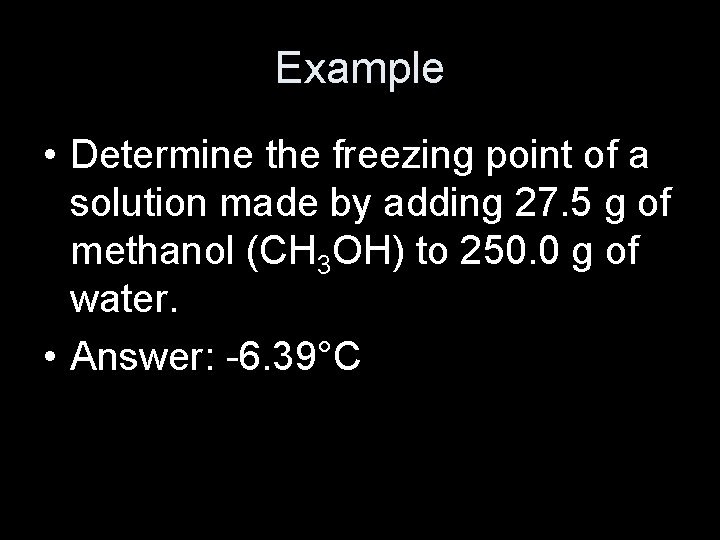Example • Determine the freezing point of a solution made by adding 27. 5 g of methanol (CH 3 OH) to 250. 0 g of water. • Answer: -6. 39°C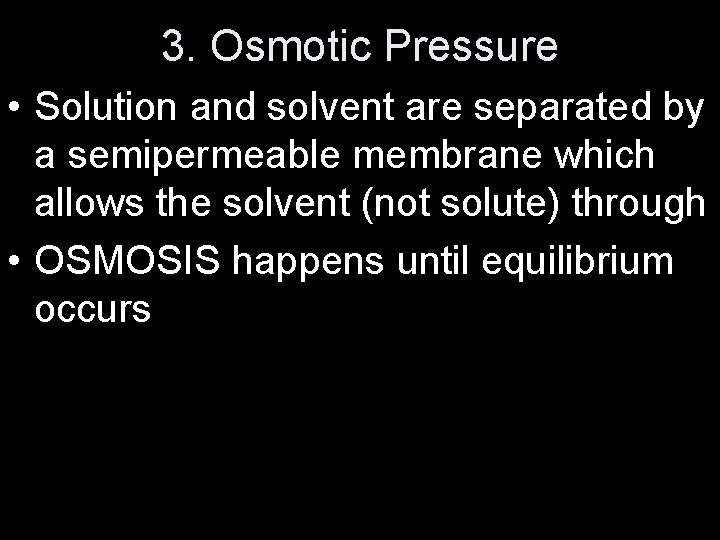3. Osmotic Pressure • Solution and solvent are separated by a semipermeable membrane which allows the solvent (not solute) through • OSMOSIS happens until equilibrium occurs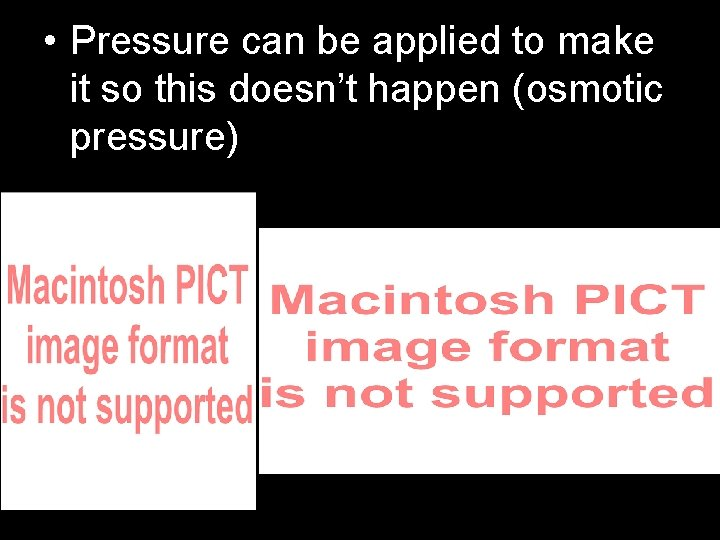• Pressure can be applied to make it so this doesn’t happen (osmotic pressure)Molar Mass From Osmotic Pressure • Useful b/c small concentration of solute can lead to a large osmotic pressure ∏ = MRT • • ∏ = osmotic pressure (atm) M = molarity of solution R = ideal gas law constant (0. 08206 Latm/Kmol) T = temperature (K)Example • The osmotic pressure of a solution containing 26. 5 mg of aspartame per liter is 1. 70 torr at 30 C. Calculate the molar mass of aspartame. • Answer: 294 g/mol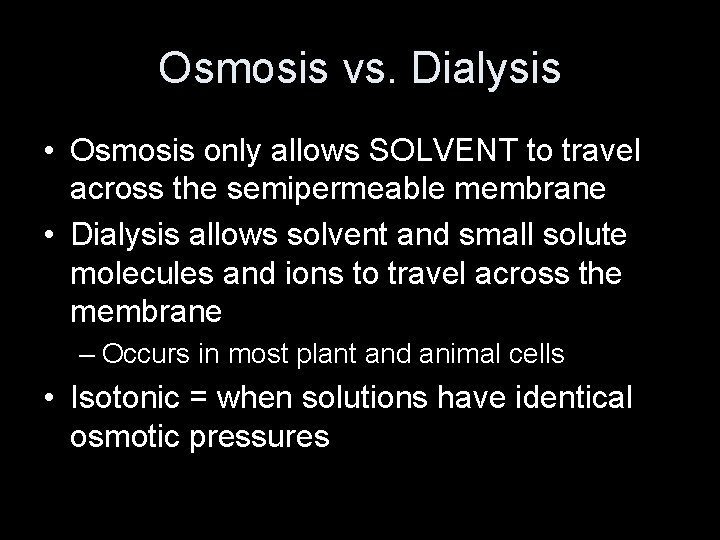Osmosis vs. Dialysis • Osmosis only allows SOLVENT to travel across the semipermeable membrane • Dialysis allows solvent and small solute molecules and ions to travel across the membrane – Occurs in most plant and animal cells • Isotonic = when solutions have identical osmotic pressures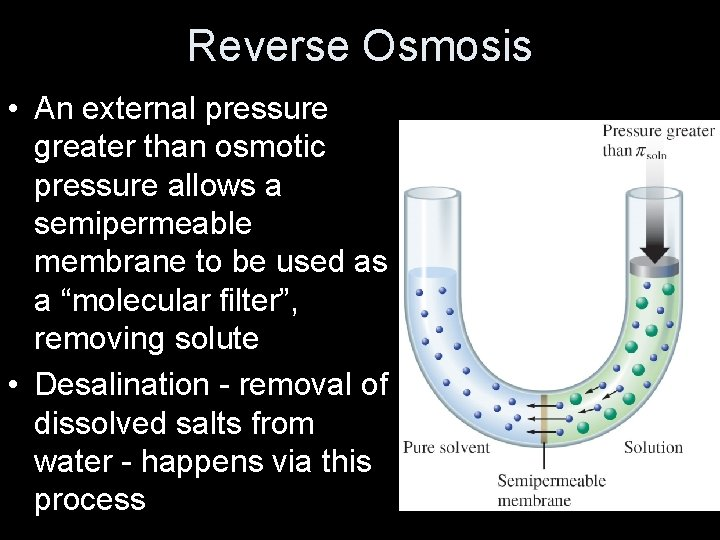Reverse Osmosis • An external pressure greater than osmotic pressure allows a semipermeable membrane to be used as a “molecular filter”, removing solute • Desalination - removal of dissolved salts from water - happens via this process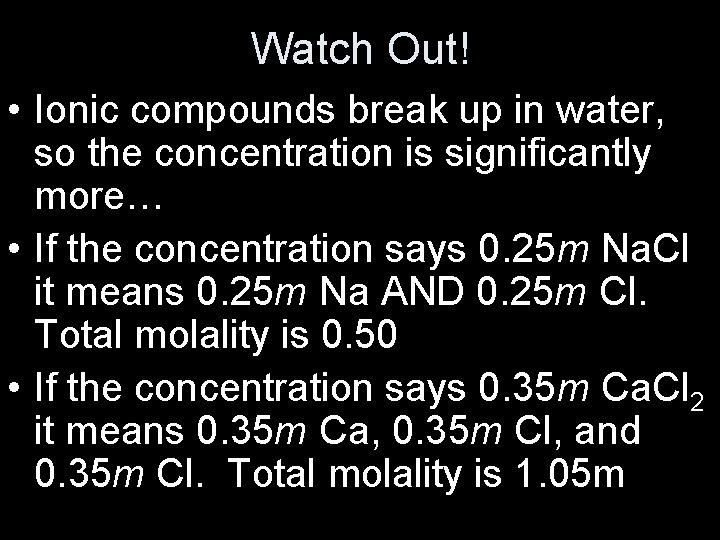Watch Out! • Ionic compounds break up in water, so the concentration is significantly more… • If the concentration says 0. 25 m Na. Cl it means 0. 25 m Na AND 0. 25 m Cl. Total molality is 0. 50 • If the concentration says 0. 35 m Ca. Cl 2 it means 0. 35 m Ca, 0. 35 m Cl, and 0. 35 m Cl. Total molality is 1. 05 mAdjustment…van’t Hoff Factor, i • i = moles of particles in solution moles of solute dissolved • This modifies the equations for freezing/boiling point changes: ∆T = im. K • Also modifies the equation for osmotic pressure: ∏ = i. MRTExample • Find the boiling point of a 1. 50 m solution of calcium chloride, Ca. Cl 2 and water. • Answer: 2. 30°C so the new boiling point would be 102. 30°C.Example - WATCH OUT! • Use data from Table 11. 6 in your textbook to calculate the freezing point and expected osmotic pressure of a 0. 50 m Fe. Cl 3 solution at 25. 0 C. (Assume the density of the final solution equals 1. 0 g/m. L and that the liquid volume is unchanged by the addition of Fe. Cl 3. ) • Answer: FP: -0. 32 C, OP: 4. 16 atmAdditional Definitions • Colloid/colloidal dispersion: suspension of tiny particles in a medium • Tyndall Effect: scattering of light by particles • Coagulation: destruction of a colloid through heat or electrolyte addition